# Test: Fourier Series, Numerical Methods & Complex Variables- 1

## 20 Questions MCQ Test Topicwise Question Bank for Electronics Engineering | Test: Fourier Series, Numerical Methods & Complex Variables- 1

Description
Attempt Test: Fourier Series, Numerical Methods & Complex Variables- 1 | 20 questions in 60 minutes | Mock test for Civil Engineering (CE) preparation | Free important questions MCQ to study Topicwise Question Bank for Electronics Engineering for Civil Engineering (CE) Exam | Download free PDF with solutions
QUESTION: 1

### The Fourier series of a real periodic function has only  P. cosine terms if it is even   Q. sine terms if it is even   R. cosine terms if it is odd   S. sine terms if it is odd  Which of the above statements are correct?

Solution:

Because sine function is odd and cosine is even function.

QUESTION: 2

### For the function e–x, the linear approximation around x = 2 is

Solution: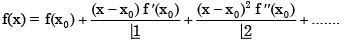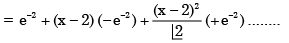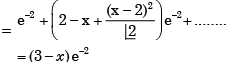(neglecting higher power of x)

QUESTION: 3

### In the Taylor series expansion of exp(x) + sin(x) about the point x = π, the coefficient of (x – π)2 is

Solution: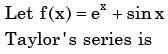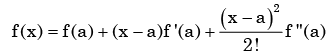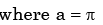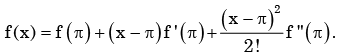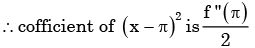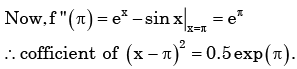QUESTION: 4

The function x(t) is shown in the figure. Even and odd parts of a unit-step function u(t) are respectively,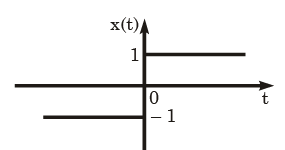Solution: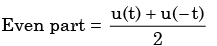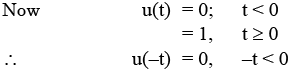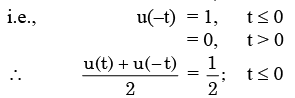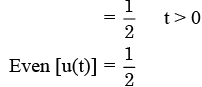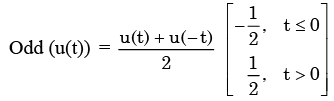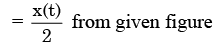QUESTION: 5

In the Taylor series expansion of ex about x = 2, the coefficient of (x - 2)4 is

Solution:

Taylor series expansion of f(x) about a is given by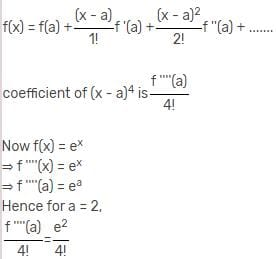QUESTION: 6

The Fourier series expansion of a symmetric and even function, f(x) where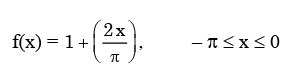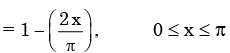Will be

Solution:

f(x) is symmetric and even, it’s Fourier series contain only cosine term.  Now.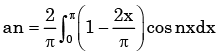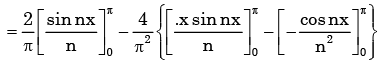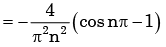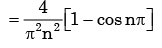QUESTION: 7

The Fourier series expansion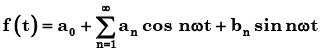of the periodic signal shown below will contain the following nonzero terms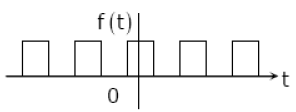Solution:

from the figure, we can say that   f(t) is an symmetric and even function of t.  as cost is even function so choice (b) is correct.

QUESTION: 8

The Fourier series for the function f(x)=sin2x is

Solution:

Here f(x ) = sin2 x is even function, hence f( x ) has no sine term.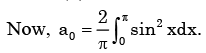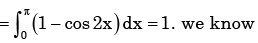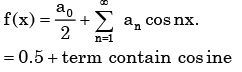QUESTION: 9

X(t) is a real valued function of a real variable with period T. Its trigonometric Fourier Series expansion contains no terms of frequency ω = 2π (2k ) /T ; k = 1, 2,.... Also, no sine terms are present. Then x(t) satisfies the equation

Solution:

No sine terms are present.

∴x(t ) is even function.

QUESTION: 10

The residue of the function f(z)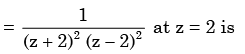Solution: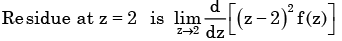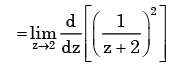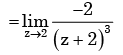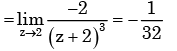QUESTION: 11

The residues of a complex function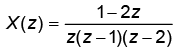​ at its poles are   ​

Solution:

x(z) has simple poles at z = 0,1, 2.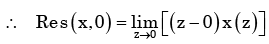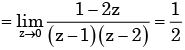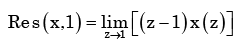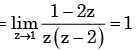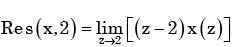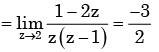QUESTION: 12

The value of the contour integral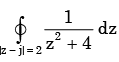in positive sense is

Solution: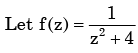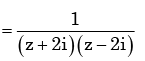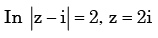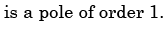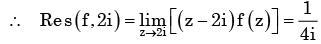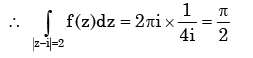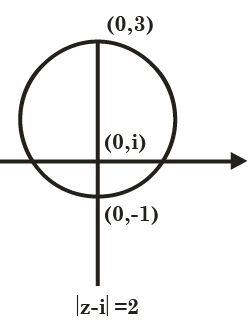QUESTION: 13

The integral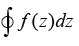evaluated around the unit circle on the complex plane for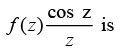Solution: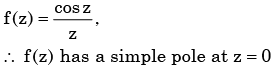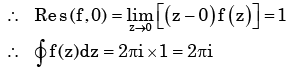QUESTION: 14

An analytic function of a complex variable z = x + iy is expressed as f(z) = u (x, y) + i v(x, y) where i =√−1 . If u = xy, the expression for v should be

Solution:

Here u and v are  analytic  as f(z) is analytic.

∴ u, v satisfy Cauchy-Riemann equation.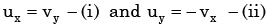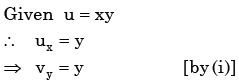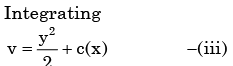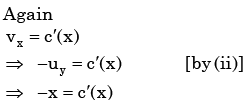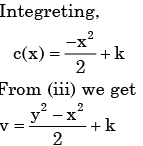QUESTION: 15

The analytic function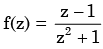has singularities at

Solution: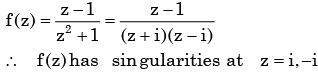QUESTION: 16

The value of the integral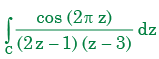(where C is a closed curve given by |z| = 1) is

Solution: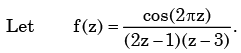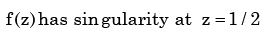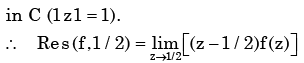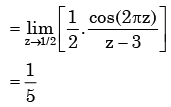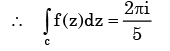QUESTION: 17

Roots of the algebraic equation x3 +x2 +x+ 1 = 0 are

Solution: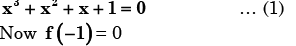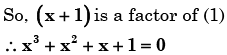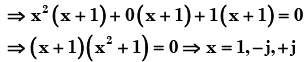QUESTION: 18

The algebraic equation

F (s ) = s5 − 3s4+ 5s3− 7s2 + 4s + 20 is given F ( s ) = 0 has

Solution: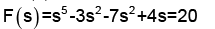we can solve it by making Routh Hurwitz array.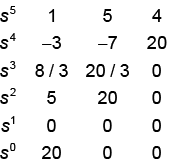We can replace 1st element of s1 by 10.

If we observe the 1st column, sign is changing two times.

So we have two poles on right half side of imaginary

Axis and 5s2+20=0

So, s =±2j and1 pole on left side of imaginary axis .

QUESTION: 19

The value of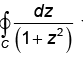where C is the contour z −i / 2=1 is

Solution:
QUESTION: 20

Let z3 = z, where z is a complex number not equal to zero. Then z is a solution of

Solution: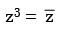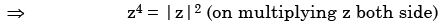Now by hit and trial method we see the solution being

Z4 = 1Use Code STAYHOME200 and get INR 200 additional OFF Use Coupon Code